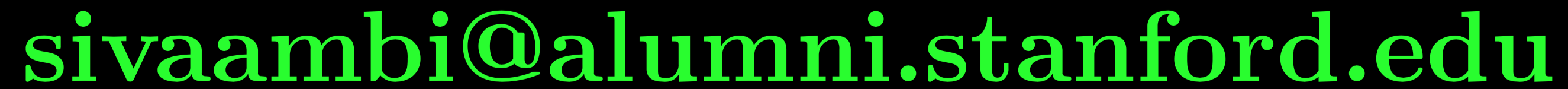# Probability, Statistics and Stochastic Processes, January-May 2019

When Tuesday 1-2, Thursday 11-12, Friday 10-11; (Monday 8-9 will be used as a reserve slot)
Where Class Room Complex 305

### Instructor

Sivaram Ambikasaran,Office: NAC 648

### Problem Sets

Problem Set 1 Problem Set 2 Problem Set 3 Problem Set 4 Problem Set 5

### Syllabus

Probability Axiomatic definition of probability, Independent Events, Baye's theorem, Discrete and continuous random variables, Distribution, Functions of random variables, Expectation, Variance, Correlation coefficient, Chebyshev’s inequality, Markov inequality, Conditional expectations, Standard discrete and continuous distributions (Binomial, Poisson, Geometric, Exponential, Normal, Chi-square), Moment generating function, Sums of random variables, Law of large numbers, Central limit theorem, Normal approximation to Binomial.
Statistics Sampling distribution, Estimation of parameters, Maximum likelihood estimates, Confidence intervals, Student’s t-distribution, Testing hypothesis, Goodness of fit.
Stochastic Processes Discrete random process, Stationary random process, Bernoulli process, Poisson process, Markov chain.

### Textbooks

• Introduction to Probability, by Dimitri Bertsekas and John N. Tsitsiklis (referred as BT in Calendar)

### Other Reference Textbooks

• Introductory Probability and Statistical Applications, by Paul L. Meyer (referred as PLM in Calendar)
• John E. Freund's Mathematical Statistics with Applications, by Irwin Miller and Marylees Miller (referred as M$$^2$$ in Calendar)
• Fundamentals of Applied Probability and Random Processes, by Oliver C. Ibe (referred as Ibe in Calendar)

 Evaluation Quiz-I Quiz-II EndSem Points 20 20 60

Quiz I will be on February $$18$$, Monday.

Quiz II will be on March $$25$$, Monday.

End Sem will be on April $$29$$, Monday.

### Calendar

Below is a very rough calendar, which will be updated as we progress through the course.
All the chapters are from BT unless indicated explicitly. Hypothesis testing will be covered from PLM.
From the book, we will be omitting Chapter 8 and Sections 5.3, 5.5, 7.5, 9.2, 9.3 and 9.4.
 Week Monday Tuesday Thursday Friday Practice problems Jan 14- Jan 18 First class- Introduction Chapter 1, BT Jan 21- Jan 25 No Class Chapter 1, BT Chapter 1, BT No Class Jan 28- Feb 1 Chapter 1, BT Chapter 1, BT Chapter 2, BT Chapter 2, BT Feb 4- Feb 8 No Class Chapter 2, BT Chapter 2, BT Chapter 2, BT Feb 11- Feb 15 No Class Chapter 3, BT Chapter 3, BT Chapter 3, BT Feb 18- Feb 22 QUIZ-I No Class No Class No Class Feb 25- Mar 1 Chapter 3, BT Chapter 3, BT No Class Chapter 3, BT Mar 4- Mar 8 Chapter 4, BT Chapter 4, BT Chapter 4, BT No Class Mar 11- Mar 15 No Class Chapter 4, 5, BT Chapter 5, BT Chapter 5, BT Mar 18- Mar 22 Chapter 5, 9, BT Section 9.1, BT Section 9.1, BT Mar 25- Mar 29 QUIZ-II Section 9.1, BT Section 9.1, BT Hypothesis Testing, PLM Apr 1- Apr 5 Hypothesis Testing, PLM Hypothesis Testing, PLM Chapter 6, BT Apr 8- Apr 12 Chapter 6, BT Chapter 6, BT Finish Chapter 6, BT Apr 15- Apr 19 Chapter 7, BT Chapter 7, BT Chapter 7, BT Holiday Apr 22- Apr 26 Chapter 7 Chapter 7 Finish Chapter 7 Apr 29- May 3 END SEM